DraftKings Championship Odds
+800
8 to 1Duke
6.5% implied probability

No changes have been recorded yet.
+800
8 to 1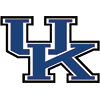Kentucky
6.5% implied probability

No changes have been recorded yet.
+900
9 to 1Michigan State
5.8% implied probability

+900
9 to 1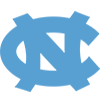North Carolina
5.8% implied probability

+1100
11 to 1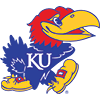Kansas
4.8% implied probability

+1400
14 to 1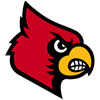Louisville
3.9% implied probability

+1600
16 to 1Memphis
3.4% implied probability

No changes have been recorded yet.
+1800
18 to 1Virginia
3.1% implied probability

+1800
18 to 1Gonzaga
3.1% implied probability

No changes have been recorded yet.
+2000
20 to 1Villanova
2.8% implied probability

No changes have been recorded yet.
+2000
20 to 1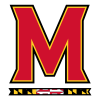Maryland
2.8% implied probability

+2000
20 to 1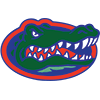Florida
2.8% implied probability

No changes have been recorded yet.
+2800
28 to 1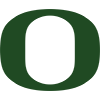Oregon
2.0% implied probability

No changes have been recorded yet.
+3300
33 to 1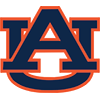Auburn
1.7% implied probability

No changes have been recorded yet.
+3300
33 to 1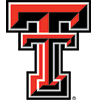Texas Tech
1.7% implied probability

No changes have been recorded yet.
+4000
40 to 1Texas
1.4% implied probability

No changes have been recorded yet.
+4000
40 to 1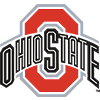Ohio State
1.4% implied probability

No changes have been recorded yet.
+4000
40 to 1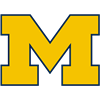Michigan
1.4% implied probability

+4000
40 to 1St. Mary's (CAL)
1.4% implied probability

+4000
40 to 1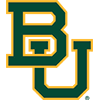Baylor
1.4% implied probability

No changes have been recorded yet.
+4000
40 to 1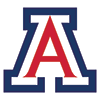Arizona
1.4% implied probability

No changes have been recorded yet.
+4000
40 to 1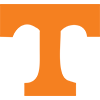Tennessee
1.4% implied probability

No changes have been recorded yet.
+5000
50 to 1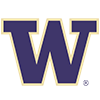Washington
1.1% implied probability

No changes have been recorded yet.
+5000
50 to 1Providence
1.1% implied probability

+5000
50 to 1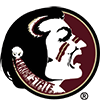Florida State
1.1% implied probability

No changes have been recorded yet.
+6000
60 to 1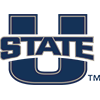Utah State
1.0% implied probability

+6600
66 to 1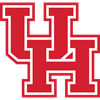Houston
0.9% implied probability

No changes have been recorded yet.
+6600
66 to 1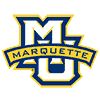Marquette
0.9% implied probability

+6600
66 to 1Georgetown
0.9% implied probability

+6600
66 to 1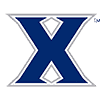Xavier
0.9% implied probability

+7000
70 to 1Wichita State
0.8% implied probability

+8000
80 to 1Wisconsin
0.7% implied probability

No changes have been recorded yet.
+8000
80 to 1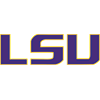Louisiana State
0.7% implied probability

+8000
80 to 1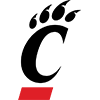Cincinnati
0.7% implied probability

No changes have been recorded yet.
+8000
80 to 1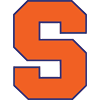Syracuse
0.7% implied probability

No changes have been recorded yet.
+8000
80 to 1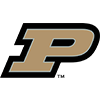Purdue
0.7% implied probability

+8000
80 to 1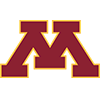Minnesota
0.7% implied probability

+8000
80 to 1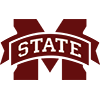Mississippi State
0.7% implied probability

+10000
100 to 1West Virginia
0.6% implied probability

No changes have been recorded yet.
+10000
100 to 1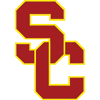USC
0.6% implied probability

+10000
100 to 1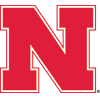Nebraska
0.6% implied probability

No changes have been recorded yet.
+10000
100 to 1Utah
0.6% implied probability

No changes have been recorded yet.
+10000
100 to 1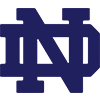Notre Dame
0.6% implied probability

+10000
100 to 1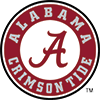Alabama
0.6% implied probability

No changes have been recorded yet.
+10000
100 to 1Missouri
0.6% implied probability

No changes have been recorded yet.
+10000
100 to 1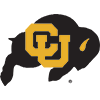Colorado
0.6% implied probability

No changes have been recorded yet.
+10000
100 to 1Iowa
0.6% implied probability

+10000
100 to 1Connecticut
0.6% implied probability

No changes have been recorded yet.
+10000
100 to 1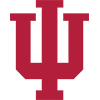Indiana
0.6% implied probability

No changes have been recorded yet.
+10000
100 to 1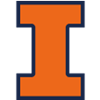Illinois
0.6% implied probability

+10000
100 to 1Creighton
0.6% implied probability

+10000
100 to 1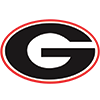Georgia
0.6% implied probability

No changes have been recorded yet.
+12000
120 to 1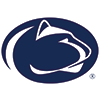Penn State
0.5% implied probability

+12000
120 to 1Arizona State
0.5% implied probability

No changes have been recorded yet.
+12000
120 to 1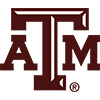Texas A&M
0.5% implied probability

No changes have been recorded yet.
+12000
120 to 1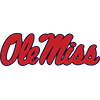Mississippi
0.5% implied probability

No changes have been recorded yet.
+12000
120 to 1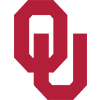Oklahoma
0.5% implied probability

No changes have been recorded yet.
+12000
120 to 1North Carolina State
0.5% implied probability

No changes have been recorded yet.
+12500
125 to 1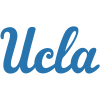UCLA
0.5% implied probability

No changes have been recorded yet.
+12500
125 to 1VCU
0.5% implied probability

No changes have been recorded yet.
+15000
150 to 1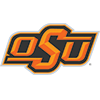Oklahoma State
0.4% implied probability

No changes have been recorded yet.
+15000
150 to 1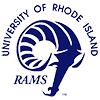Rhode Island
0.4% implied probability

No changes have been recorded yet.
+15000
150 to 1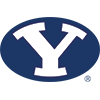BYU
0.4% implied probability

No changes have been recorded yet.
+20000
200 to 1Clemson
0.3% implied probability

No changes have been recorded yet.
+20000
200 to 1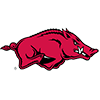Arkansas
0.3% implied probability

+20000
200 to 1Kansas State
0.3% implied probability

+20000
200 to 1Butler
0.3% implied probability

+20000
200 to 1Virginia Tech
0.3% implied probability

No changes have been recorded yet.
+20000
200 to 1Wake Forest
0.3% implied probability

No changes have been recorded yet.
+20000
200 to 1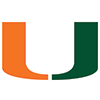Miami
0.3% implied probability

No changes have been recorded yet.
+20000
200 to 1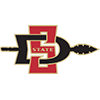San Diego State
0.3% implied probability

No changes have been recorded yet.
+20000
200 to 1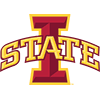Iowa State
0.3% implied probability

+22500
225 to 1Boise State
0.3% implied probability

No changes have been recorded yet.
+22500
225 to 1Davidson
0.3% implied probability

No changes have been recorded yet.
+25000
250 to 1Vanderbilt
0.2% implied probability

No changes have been recorded yet.
+25000
250 to 1Loyola-Chicago
0.2% implied probability

No changes have been recorded yet.
+25000
250 to 1Oregon State
0.2% implied probability

No changes have been recorded yet.
+25000
250 to 1UNLV
0.2% implied probability

No changes have been recorded yet.
+25000
250 to 1Boston College
0.2% implied probability

No changes have been recorded yet.
+25000
250 to 1Temple
0.2% implied probability

No changes have been recorded yet.
+25000
250 to 1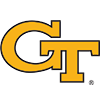Georgia Tech
0.2% implied probability

No changes have been recorded yet.
+25000
250 to 1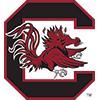South Carolina
0.2% implied probability

No changes have been recorded yet.
+25000
250 to 1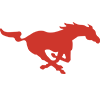SMU
0.2% implied probability

No changes have been recorded yet.
+30000
300 to 1Nevada
0.2% implied probability

No changes have been recorded yet.
+30000
300 to 1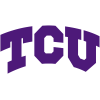TCU
0.2% implied probability

No changes have been recorded yet.
+30000
300 to 1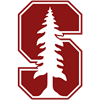Stanford
0.2% implied probability

No changes have been recorded yet.
+30000
300 to 1St. John's
0.2% implied probability

+30000
300 to 1Saint Louis
0.2% implied probability

No changes have been recorded yet.
+30000
300 to 1New Mexico State
0.2% implied probability

No changes have been recorded yet.
+30000
300 to 1New Mexico
0.2% implied probability

No changes have been recorded yet.
+30000
300 to 1Missouri State
0.2% implied probability

No changes have been recorded yet.
+50000
500 to 1Dayton
0.1% implied probability

No changes have been recorded yet.